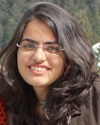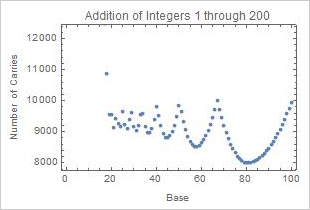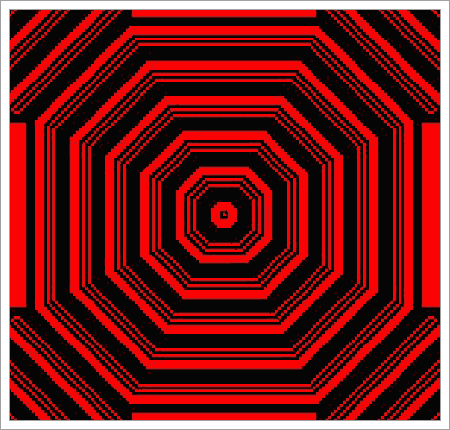#Wolfram Summer School

# Alumni# Mugdha Thakur

Summer School

Class of 2014

## Bio

I am an Int. MS (Mathematics) student at the Indian Institute of Science Education and Research (IISER), Mohali. My major interests lie in probability and statistics, programming, and evolutionary theory. Apart from this, I like to read about the history of numbers, number systems, etc., and observing changes and patterns. My hobbies are reading and dancing.

## Project: The Complexity of Arithmetic in Different Bases on a Finite Set of Inputs

Starting with integer bases, algorithms for addition, multiplication, and division are used to perform operations on a finite set of pairs of numbers to determine the comparitive efficiency of these operations in many bases. The efficiency of addition and multiplication is measured by counting the number of carries in the traditional arithmetic algorithms. For division, the length of the repetend (that is, the length of the repeating sequence of digits to the right of the radix point) is used as a measure of efficiency. Several mixed base systems are also studied, including the factorial number system, the imperial length system, and the English currency system.## Favorite Outer Totalistic r=1, k=2 2D Cellular Automaton

Rule 65189• 21st Annual Wolfram Summer School
• Bentley University, Waltham, MA, USA
• June 25–July 15, 2023